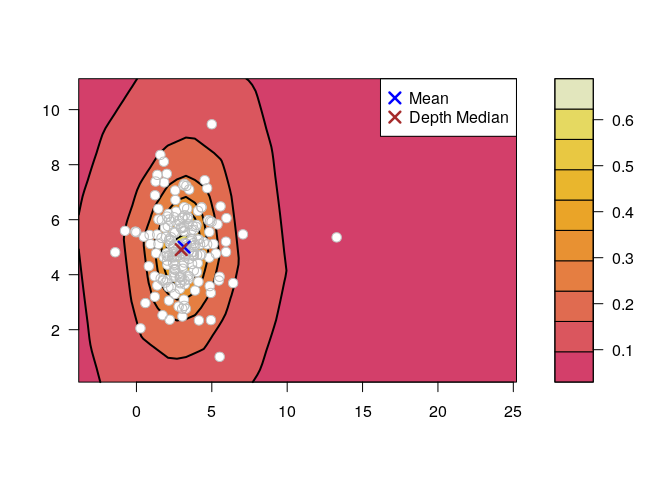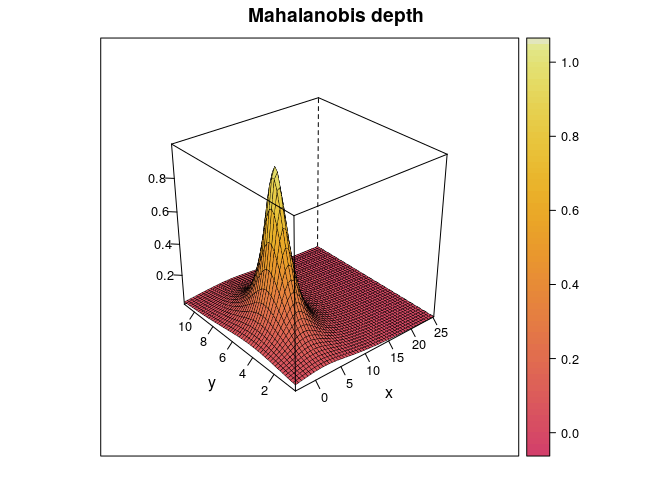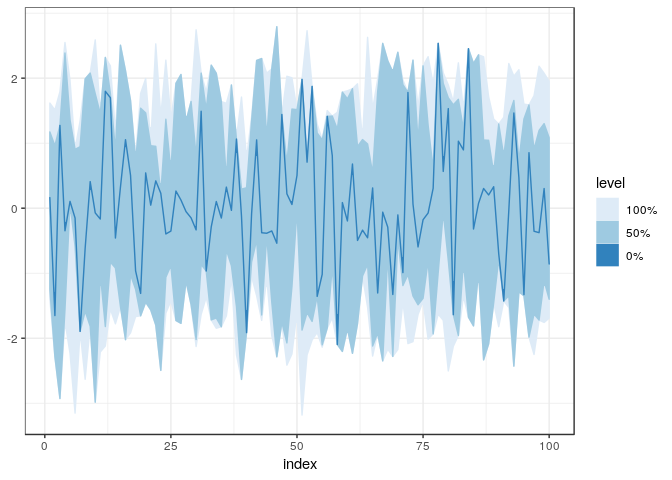DepthProc project consist of a set of statistical procedures based on so called statistical depth functions. The project involves free available R package and its description.

## Installation

DepthProc is avaiable on CRAN:

install.packages("DepthProc")

You can also install it from GitHub with devtools package:

library(devtools)
install_github("zzawadz/DepthProc")

## Main features:

Most of the code is written in C++ for additional efficiency. We also use OpenMP to speedup computations with multithreading:

library(DepthProc)
set.seed(123)

d <- 10
x <- mvrnorm(1000, rep(0, d), diag(d))
# Default - utilize as many threads as possible
system.time(depth(x, x, method = "LP"))
#>    user  system elapsed
#>   0.351   0.000   0.090

# Only single thread - 4 times slower:
system.time(depth(x, x, method = "LP", threads = 1))
#>    user  system elapsed
#>   0.208   0.000   0.208

# Two threads - 2 times slower:
system.time(depth(x, x, method = "LP", threads = 2))
#>    user  system elapsed
#>   0.201   0.000   0.103

## Available depth functions

x <- mvrnorm(100, c(0, 0), diag(2))

depthEuclid(x, x)
depthMah(x, x)
depthLP(x, x)
depthProjection(x, x)
depthLocal(x, x)
depthTukey(x, x)

## Base function to call others:
depth(x, x, method = "Projection")
depth(x, x, method = "Local", depth_params1 = list(method = "LP"))

## Get median
depthMedian(x,
depth_params = list(
method = "Local",
depth_params1 = list(method = "LP")))

## Basic plots

### Contour plot

library(mvtnorm)
y <- rmvt(n = 200, sigma = diag(2), df = 4, delta = c(3, 5))
depthContour(y, points = TRUE, graph_params = list(lwd = 2))### Perspective plot

depthPersp(y, depth_params = list(method = "Mahalanobis"))## Functional depths:

There are two functional depths implemented - modified band depth (MBD), and Frainman-Muniz depth (FM):

x <- matrix(rnorm(60), nc = 20)
fncDepth(x, method = "MBD")
fncDepth(x, method = "FM", dep1d = "Mahalanobis")
#> Warning in dep1d_params\$u <- u[, i]: Coercing LHS to a list

### Functional BoxPlot

x <- matrix(rnorm(2000), ncol = 100)
fncBoxPlot(x, bands = c(0, 0.5, 1), method = "FM")